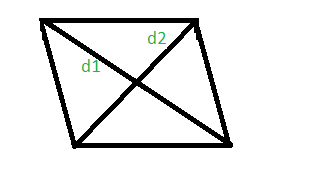Open In App

# Program to calculate area and perimeter of a rhombus whose diagonals are given

Given the length of diagonals of a rhombus, d1 and d2. The task is to find the perimeter and the area of that rhombus.
A rhombus is a polygon having 4 equal sides in which both the opposite sides are parallel, and opposite angles are equal.Examples:

Input: d1 = 2 and d2 = 4
Output: The area of rhombus with diagonals 2 and 4 is 4.
The perimeter of rhombus with diagonals 2 and 4 is 8.

Input: d1 = 100 and d2 = 500
Output: The area of rhombus with diagonals 100 and 500 is 25000.
The perimeter of rhombus with diagonals 100 and 500 is 1019.

Area of the rhombus =Perimeterof the rhombus =Below is the implementation of above approach:

## C++

 // C++ Program to calculate area and perimeter// of a rhombus using diagonals#include #include using namespace std; // calculate area and perimeter of a rhombusint rhombusAreaPeri(int d1, int d2){    long long int area, perimeter;     area = (d1 * d2) / 2;    perimeter = 2 * sqrt(pow(d1, 2) + pow(d2, 2));     cout << "The area of rhombus with diagonals "         << d1 << " and " << d2 << " is " << area << "." << endl;     cout << "The perimeter of rhombus with diagonals "         << d1 << " and " << d2 << " is " << perimeter << "." << endl;} // Driver codeint main(){     int d1 = 2, d2 = 4;    rhombusAreaPeri(d1, d2);     return 0;}

## Java

 // Java program to calculate area and perimeter// of a rhombus using diagonalsimport java.io.*; class GFG {   // calculate area and perimeter of a rhombusstatic int rhombusAreaPeri(int d1, int d2){     int area, perimeter;     area = (d1 * d2) / 2;    perimeter = (int)(2 * Math.sqrt(Math.pow(d1, 2) + Math.pow(d2, 2)));     System.out.println( "The area of rhombus with diagonals "        + d1 + " and " + d2 + " is " + area + ".");     System.out.println("The perimeter of rhombus with diagonals "        +d1 + " and " + d2 + " is " + perimeter + ".");        return 0;} // Driver code      public static void main (String[] args) {        int d1 = 2, d2 = 4;    rhombusAreaPeri(d1, d2);    }}// This code is contributed by anuj_67..

## Python3

 # Python 3 Program to calculate# area and perimeter of a rhombus# using diagonalsfrom math import sqrt, pow # calculate area and perimeter# of a rhombusdef rhombusAreaPeri(d1, d2):    area = (d1 * d2) / 2    perimeter = 2 * sqrt(pow(d1, 2) +                         pow(d2, 2))     print("The area of rhombus with diagonals",                d1, "and", d2, "is", area, ".")     print("The perimeter of rhombus with diagonals",                d1, "and", d2, "is", perimeter, "." ) # Driver codeif __name__ == '__main__':    d1 = 2    d2 = 4    rhombusAreaPeri(d1, d2) # This code is contributed# by Surendra_Gangwar

## C#

 // C# program to calculate area// and perimeter of a rhombus// using diagonalsusing System; class GFG{ // calculate area and perimeter// of a rhombusstatic int rhombusAreaPeri(int d1,                            int d2){    int area, perimeter;     area = (d1 * d2) / 2;    perimeter = (int)(2 * Math.Sqrt(Math.Pow(d1, 2) +                                    Math.Pow(d2, 2)));     Console.WriteLine( "The area of rhombus with " +                       "diagonals " + d1 + " and " +                          d2 + " is " + area + ".");     Console.WriteLine("The perimeter of rhombus " +                      "with diagonals " + d1 + " and " +                         d2 + " is " + perimeter + ".");        return 0;} // Driver codepublic static void Main (){    int d1 = 2, d2 = 4;    rhombusAreaPeri(d1, d2);}} // This code is contributed by anuj_67..

## PHP

 

## Javascript

 

Output:

The area of rhombus with diagonals 2 and 4 is 4.
The perimeter of rhombus with diagonals 2 and 4 is 8.

Time Complexity: O(log(d12+d22))
Auxiliary Space: O(1), since no extra space has been taken.